Timer ICs / ASSPs

# What is intermittent operation?

Intermittent operation is a means of substantially lowering the power consumption of IoT communication devices and monitoring devices.
In the following, we will describe the relationship between intermittent operation and current consumption, and introduce examples of circuits that reduce power consumption through intermittent operation.

## 1.What is intermittent operation?

While intermittent operation may be an unfamiliar term, in fact is a technology widely used in devices around us.

We associate “intermittent” with intermittent windshield wipers, geysers, or similar phenomena that occur at intervals.“Intermittent operation” is a term frequently used in the field of electric control. As a system of operation control, it refers to a method of control whereby normal and standby operations are regularly repeated.

For example, normal operation of battery-powered and energy-harvesting devices or other systems with a limited supply of power results in current consumption, rapidly depleting battery power.
For this reason, the majority of such devices use “intermittent operation” to regularly go into standby mode—reducing current consumption and using available power sources efficiently.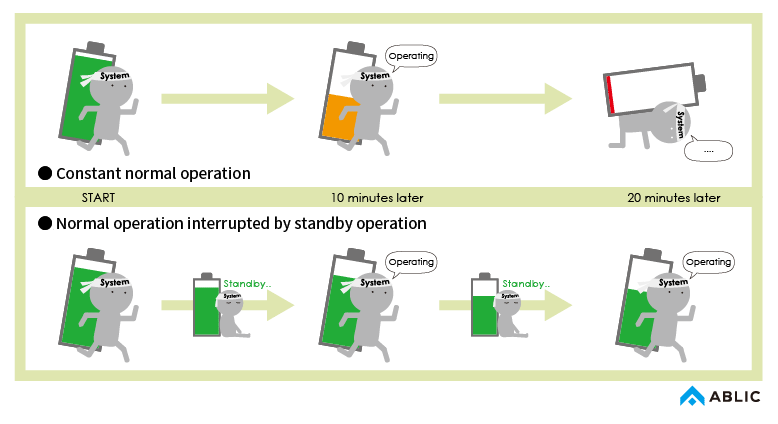Intermittent operation is a very effective means of reducing current consumption in systems that permit regular standby operation (IoT communication devices and monitoring devices).

## 2. Current consumption in intermittent operation

The following provides a detailed description of the relationship between intermittent operation and current consumption.

As shown below, in intermittent operation, normal operation and standby operation are repeated in the system to reduce average current consumption.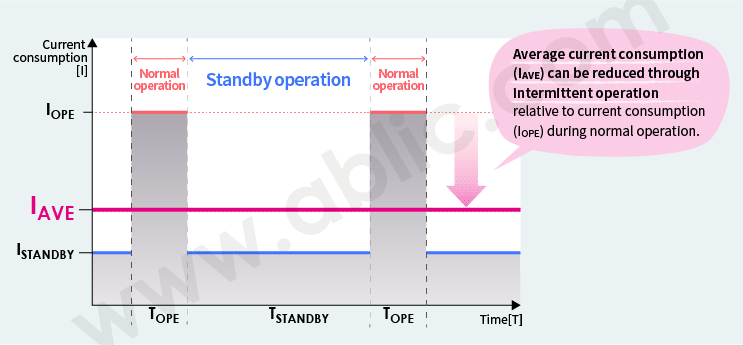IOPE＝current consumption during normal operation
ISTANDBY=current consumption during standby operation
IAVE=average current consumption
TOPE=normal operation time
TSTANDBY=standby operation time

The following equation is used to calculate average current consumption (IAVE).

IAVE

IOPE × TOPE + ISTANDBY × TSTANDBY

TOPE + TSTANDBY

For example, in intermittent operation (TOPE = 0.1sec, TSTANDBY = 0.9sec) where normal operation is performed for only 0.1sec per second under the conditions of IOPE = 10mA and ISTANDBY = 10μA (=0.01mA), we obtain the result shown in the following equation.

IAVE

10mA × 0.1sec + 10μA × 0.9sec

0.1sec + 0.9sec

= 1.009mA ≒ 1mA

This means that in intermittent operation current consumption is 1/10 of the current consumption under normal operation. Effective reduction in current consumption of a system is proportional to the ratio of intermittent operation spent in standby operation.

## 3. Example of intermittent operation(1) – Example of circuit running on an MCU

A microcontroller unit (MCU)* is often used in intermittent operation as a trigger for switching the overall system between normal operation and standby operation.

*Microcontroller･･･ A compact computer for controlling electronic devices. As the brain of electronic devices, MCUs operate according to input signals.

An MCU programmed to switch from standby to normal operation at specified periods can be used to control system intermittent operation.

For example, in intermittent operation (TOPE = 0.1sec, and TSTANDBY = 3599.9sec) where normal operation is performed for only 0.1sec per hour under the following conditions of IOPE = 10mA, LDO ISTANDBY = 5μA and MCU ISTANDBY (in sleep mode) = 5 μA, we obtain the result shown in the following equation.

IAVE

10mA × 0.1sec + (5μA + 5μA) × 3599.9sec

0.1sec + 3599.9sec

= 10.3μA ≒ 10μA

As shown in the following figure, the average current consumption (IAVE) is reduced to about the same degree as current consumption during standby operation (ISTADNBY).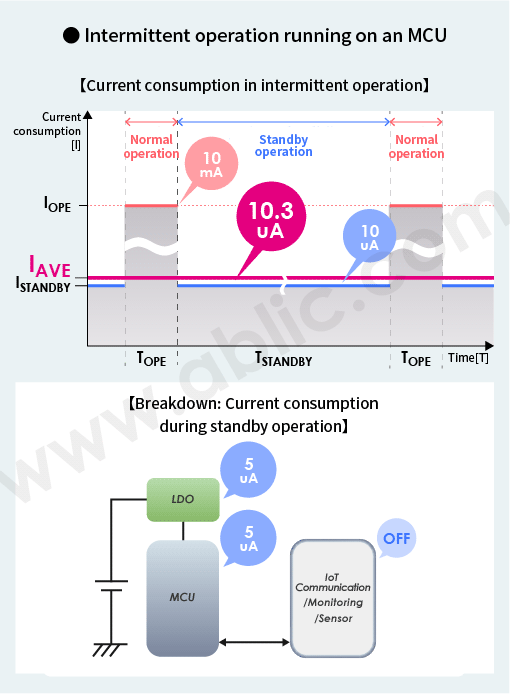## 4.Example of intermittent operation (2) – Example of an ultra-low current consumption circuit using a timer IC

To reduce current consumption below that shown in “3. Example of intermittent operation (1)” above, follow the procedure below:

• Select a low power consumption LDO
• Select an MCU with low current consumption in sleep mode

Another effective approach is to

• Turn the MCU completely OFF and use a low current consumption timer IC to function as a wakeup trigger

Install a switch between the LDO and the MCU, as shown in the figure on the right, whose ON/OFF states are controlled by a timer IC that have previously been programmed to regularly wake up the system.

Since the current consumption of such a timer IC normally is lower than the current consumption of an MCU in sleep mode, this contributes to further reduction of average current consumption.

Let’s calculate the average current consumption when a timer IC is used.
Assume that the S-35710 is used as the timer IC.

Assume that IOPE = 10mA and LDO ISTANDBY = 5uA. Since the MCU is not in sleep mode, it can be turned OFF completely, ISTANDBY for the MCU is 0uA.
In intermittent operation (TOPE = 0.1sec, TSTANDBY = 3599.9sec) where normal operation is performed for only 0.1sec per hour and ISTANDBY of the S-35710 timer IC is 0.2uA, we obtain the result shown in the following equation.

IAVE

10mA × 0.1sec + (5μA + 0.2μA) × 3599.9sec

0.1sec + 3599.9sec

= 5.5μA

Compared to intermittent operation using MCU sleep mode introduced in “3. Example of intermittent operation (1)”, average current consumption is reduced by half.

## 5. Selecting an IC suitable for intermittent operation

As stated earlier, to use intermittent operation to reduce current consumption,

• Select an MCU with low current consumption in sleep mode.
• Use a low power consumption timer IC as a wakeup trigger to turn the MCU completely OFF during standby operation.
• LDOs are always on both during standby and normal operation. Select one with low power consumption to build a product with the required low-power specifications.

### ABLIC’s ICs use intermittent operation to support ultra-low power consumption

ABLIC provides a lineup of timer ICs and LDOs that enable intermittent operation.
Especially, combining the S-35710C01, wake-up timer IC, and the S-1318, LDO, will contribute to substantially lower system current consumption.

The current consumption of the S-35710C01 and the S-1318 is 0.2uA and 0.095uA, respectively. When the conditions described in “4. Example of intermittent operation (2)” apply, we obtain the result shown in the following equation.
When the conditions described in “4. Example of intermittent operation (2)”* apply, we obtain the result shown in the following equation.

*In intermittent operation (TOPE = 0.1sec, TSTANDBY = 3599.9sec) where normal operation is performed for only 0.1sec per hour

IAVE

10mA × 0.1sec + (0.095μA + 0.2μA) × 3599.9sec

0.1sec + 3599.9sec

= 0.57μA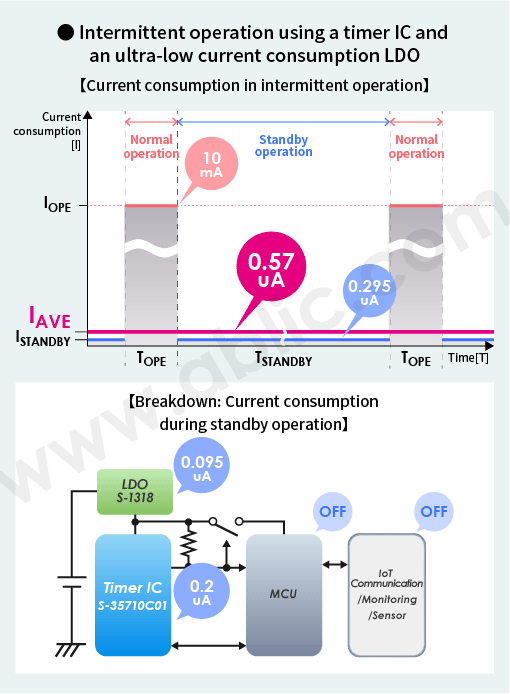In addition, ABLIC provides a lineup of low power consumption wake-up timer ICs and interval timer ICs that enable flexible time settings to support intermittent operation. These products support the development of IoT communication devices, monitoring devices, security devices, and a wide variety of other battery-operated systems or energy harvesting systems.

If your goal is to build a device with low current consumption through intermittent operation, ABLIC’s ICs are highly recommended.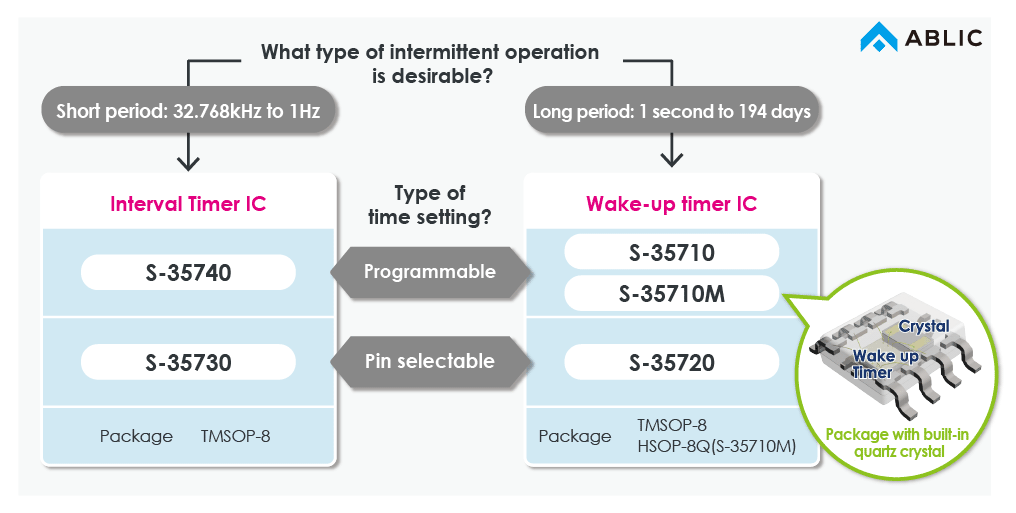S-35740

S-35730

S-35720

S-35710M

S-35710